### Samurai champlooHover over the thumbnail for a full-size version.

Author Maxtreme author:maxtreme bitesized n-art rated 2006-05-06 4 by 58 people. \$Samurai champloo#Maxtreme#none#00000000000000000000000000000000000000000000000000000000000000000000000000000000000000000000000000000000000000000000000000000000000000000000000000000000000000000000000000000000000000000000000000000000000000000000000000000000000000000000000000000000000000000000000000000000000000000000000000000000000000000000000000000000000000000000000000000000000000000000000000000000000000000000000000000000000000000000000000000000000000000000000000000000000000000000000000000000000000000000000000000000000000000000000000000000000000000000000000000000000000000000000000000000000000000000000000000000000000000000000000000000000000000000000000000000000000000000000000000000000000000000000000000000000000000000000000000000000000000|12^234,162!12^228,168!12^222,174!12^216,180!12^210,186!12^216,174!12^222,168!12^228,156!12^228,162!12^228,150!12^222,138!12^228,144!12^216,132!12^210,132!12^204,132!12^198,138!12^192,138!12^198,126!12^192,132!12^204,120!12^204,114!12^210,108!12^204,108!12^192,102!12^180,102!12^168,96!12^180,90!12^198,90!12^210,90!12^216,78!12^216,60!12^210,54!12^222,54!12^228,60!12^228,66!12^240,66!12^246,54!12^252,42!12^252,36!12^258,42!12^264,48!12^330,30!12^336,30!12^252,162!12^258,174!12^264,180!12^252,180!12^258,210!12^258,222!12^276,246!12^288,234!12^282,246!12^282,270!12^288,282!12^306,288!12^294,300!12^300,306!12^306,318!12^318,324!12^330,318!12^348,318!12^366,306!12^396,294!12^348,324!12^354,336!12^366,348!12^378,366!12^378,378!12^366,354!12^156,336!12^150,324!12^138,306!12^126,306!12^120,324!12^114,330!12^102,336!12^90,336!12^84,336!12^66,342!12^48,360!12^54,378!12^72,402!12^96,414!12^96,426!12^102,444!12^114,468!12^120,492!12^126,510!12^126,534!12^132,552!12^132,558!12^342,48!12^384,54!12^426,78!12^402,78!12^384,84!12^414,84!12^444,102!12^462,96!12^468,90!12^468,108!12^456,114!12^456,132!12^468,144!12^486,150!12^468,156!12^498,174!12^468,186!12^456,198!12^444,204!12^462,216!12^456,216!12^444,228!12^492,228!12^474,252!12^474,264!12^468,264!12^456,258!12^438,282!12^510,378!12^528,390!12^468,426!12^420,456!12^366,534!12^360,576!12^540,408!12^462,288!12^234,150!12^234,156!12^234,132!12^222,126!12^216,126!12^210,126!12^192,138!12^198,126!12^198,132!12^204,126!12^210,120!12^210,114!12^240,108!12^246,108!12^282,114!12^258,90!12^234,90!12^234,90!12^222,96!12^216,96!12^204,96!12^204,96!12^198,102!12^192,96!12^180,96!12^168,96!12^174,96!12^186,96!12^186,90!12^186,102!12^192,90!12^204,96!12^204,96!12^198,96!12^210,96!12^216,102!12^210,102!12^204,102!12^228,102!12^216,84!12^264,240!12^270,246!12^258,240!12^258,234!12^300,216!12^300,234!12^300,252!12^288,270!12^240,168!12^252,174!12^252,174!12^246,168!12^240,156!12^240,162!12^252,162!12^276,144!12^270,192!12^288,234!12^276,228!12^276,228!12^276,216!12^276,204!12^276,198!12^276,192!12^282,210!12^276,210!12^282,222!12^276,222!12^282,216!12^288,216!12^288,222!12^294,228!12^294,222!12^294,216!12^282,228!12^288,228!12^258,204!12^258,186!12^258,216!12^258,228!12^276,240!12^276,234!12^270,222!12^270,204!12^276,198!12^270,198!12^270,216!12^276,252!12^276,264!12^270,270!12^276,270!12^348,330!12^360,342!12^372,360!12^378,372!12^216,66!12^228,66!12^228,84!12^222,78!12^222,72!12^222,66!12^222,66!12^222,60!12^222,84!12^228,90!12^222,90!12^216,90!12^288,240!12^282,240!12^282,234!12^300,240!12^294,252!12^294,264!12^300,246!12^288,294!12^306,294!12^300,294!12^294,294!12^306,324!12^312,324!12^324,318!12^324,318!12^318,312!12^318,312!12^306,306!12^300,300!12^312,300!12^318,318!12^312,318!12^306,300!12^312,306!12^312,312!12^306,312!12^276,258!12^342,306!12^348,300!12^336,312!12^336,300!12^342,294!12^348,288!12^348,312!12^360,306!12^354,312!12^330,306!12^324,312!12^324,306!12^324,288!12^324,264!12^324,240!12^330,216!12^300,258!12^300,264!12^294,270!12^306,270!12^300,276!12^306,270!12^282,282!12^150,348!12^144,336!12^138,324!12^132,318!12^156,360!12^156,372!12^156,378!12^156,390!12^150,396!12^144,402!12^138,414!12^138,426!12^150,438!12^156,456!12^144,426!12^144,414!12^162,474!12^174,504!12^186,528!12^210,558!12^216,576!12^132,576!12^132,570!12^132,564!12^336,24!12^234,54!12^240,42!12^240,42!12^348,36!12^444,48!12^426,60!12^426,72!12^456,84!12^486,84!12^504,78!12^504,90!12^504,114!12^492,126!12^474,306!12^492,342!12^300,210!12^294,204!12^282,198!12^294,210!12^288,204!12^282,204!12^288,210!12^300,162!12^294,156!12^300,138!12^312,126!12^318,120!12^366,138!12^366,156!12^330,168!12^312,156!12^342,126!12^348,114!12^336,90!12^294,78!12^270,72!12^258,72!12^252,66!12^264,60!12^300,72!12^120,324!12^126,318!12^114,312!12^114,318!12^126,330!12^132,336!12^132,342!12^138,354!12^144,366!12^132,348!12^144,360!12^144,372!12^144,330!12^150,342!12^156,366!12^150,378!12^150,384!12^66,348!12^60,360!12^54,354!12^54,366!12^48,366!12^48,372!12^42,372!12^36,378!12^30,408!12^36,402!12^66,408!12^48,420!12^30,426!12^114,306!12^108,324!12^108,330!12^108,318!12^114,324!12^114,336!12^120,330!12^132,336!12^126,342!12^126,336!12^120,336!12^120,348!12^132,360!12^114,360!12^60,384!12^66,390!12^78,396!12^90,402!12^84,402!12^90,408!12^102,384!12^90,378!12^90,378!12^96,372!12^90,366!12^84,366!12^72,378!12^72,378!12^72,372!12^72,366!12^90,360!12^90,354!12^102,354!12^102,360!12^102,366!12^108,366!12^114,372!12^126,384!12^120,384!12^114,390!12^108,390!12^102,390!12^84,384!12^84,384!12^78,378!12^78,378!12^84,372!12^78,372!12^78,360!12^78,354!12^66,366!12^60,372!12^60,372!12^54,366!12^60,366!12^84,378!12^90,372!12^78,372!12^66,378!12^66,384!12^60,378!12^66,372!12^54,372!12^84,366!12^78,360!12^78,366!12^84,360!12^84,354!12^90,348!12^96,342!12^108,342!12^102,342!12^108,336!12^96,354!12^96,360!12^96,366!12^102,348!12^102,348!12^96,348!12^114,348!12^114,354!12^120,366!12^126,372!12^108,354!12^108,348!12^114,342!12^120,342!12^108,360!12^96,432!12^96,438!12^108,450!12^114,456!12^114,468!12^108,462!12^108,456!12^102,450!12^108,468!12^108,474!12^108,480!12^108,492!12^114,498!12^114,504!12^114,516!12^120,528!12^126,546!12^126,552!12^126,570!12^126,564!12^126,558!12^126,576!12^252,156!12^252,156!12^240,144!12^234,138!12^228,132!12^222,132!12^228,138!12^72,390!12^72,384!12^78,384!12^78,396!12^90,390!12^90,396!12^78,390!12^84,390!12^102,372!12^84,396!12^96,378!12^96,384!12^90,384!12^102,378!12^108,378!12^108,384!12^108,372!12^114,378!12^114,384!12^120,378!12^120,372!12^114,366!12^126,348!12^120,354!12^120,360!12^132,360!12^132,366!12^126,366!12^126,354!12^132,354!12^126,360!12^132,372!12^132,378!12^126,378!12^120,396!12^120,402!12^114,402!12^114,396!12^108,396!12^102,396!12^96,390!12^96,396!12^102,402!12^108,408!12^108,420!12^102,426!12^96,420!12^102,420!12^102,408!12^96,408!12^96,402!12^108,402!12^102,408!12^108,414!12^102,414!12^114,414!12^114,426!12^108,432!12^102,426!12^108,426!12^114,420!12^114,444!12^108,438!12^102,438!12^102,432!12^120,468!12^126,480!12^138,498!12^144,504!12^144,486!12^144,480!12^138,474!12^132,462!12^132,456!12^126,450!12^126,444!12^120,438!12^120,426!12^126,420!12^126,414!12^126,414!12^120,408!12^114,408!12^126,402!12^126,402!12^126,390!12^120,390!12^132,390!12^132,402!12^120,456!12^120,444!12^120,450!12^114,450!12^108,444!12^114,432!12^114,444!12^114,438!12^114,510!12^120,522!12^126,540!12^138,576!12^144,576!12^150,576!12^156,576!12^162,576!12^168,576!12^174,576!12^180,570!12^180,576!12^192,576!12^186,576!12^198,570!12^198,576!12^204,576!12^210,576!12^216,570!12^210,564!12^30,384!12^36,432!12^30,438!12^42,426!12^48,420!12^54,414!12^60,408!12^48,402!12^42,408!12^36,414!12^36,414!12^24,414!12^36,408!12^42,384!12^48,390!12^60,396!12^54,396!12^42,396!12^36,396!12^30,396!12^24,402!12^24,396!12^24,390!12^42,426!12^36,426!12^30,432!12^24,432!12^24,426!12^24,420!12^30,420!12^36,420!12^42,420!12^30,414!12^24,408!12^30,402!12^48,414!12^42,420!12^42,414!12^42,402!12^54,408!12^48,408!12^48,402!12^48,396!12^42,390!12^30,390!12^36,390!12^42,384!12^60,402!12^54,402!12^36,384!12^24,438!12^24,444!12^108,486!12^114,486!12^114,486!12^114,474!12^120,474!12^120,480!12^126,324!12^96,330!12^102,324!12^90,330!12^102,318!12^102,312!12^108,300!12^120,318!12^120,306!12^108,306!12^126,288!12^120,288!12^114,288!12^108,294!12^114,300!12^114,294!12^132,294!12^138,306!12^150,318!12^156,324!12^156,336!12^156,330!12^132,330!12^138,342!12^144,354!12^144,366!12^144,372!12^138,402!12^138,408!12^162,534!12^156,534!12^150,534!12^150,522!12^156,516!12^162,516!12^162,522!12^138,570!12^144,570!12^150,570!12^156,570!12^168,570!12^174,570!12^162,570!12^162,564!12^156,564!12^150,564!12^144,564!12^138,564!12^138,558!12^144,558!12^150,558!12^156,558!12^162,558!12^162,552!12^156,552!12^150,552!12^150,552!12^144,552!12^138,552!12^138,546!12^132,546!12^144,546!12^150,546!12^156,546!12^162,546!12^168,546!12^156,540!12^150,540!12^144,540!12^138,540!12^132,540!12^132,534!12^138,534!12^144,534!12^144,528!12^138,528!12^132,528!12^126,528!12^126,522!12^138,522!12^138,522!12^138,522!12^132,522!12^156,324!12^144,300!12^264,246!12^54,354!12^144,300!12^150,294!12^150,282!12^156,294!12^174,318!12^186,324!12^174,282!12^192,264!12^210,252!12^258,246!12^252,246!12^252,240!12^252,234!12^264,192!12^264,198!12^258,186!12^222,240!12^216,246!12^192,270!12^174,288!12^180,294!12^186,300!12^198,300!12^216,288!12^246,258!12^216,264!12^228,258!12^240,246!12^234,252!12^222,264!12^216,270!12^210,270!12^204,276!12^198,282!12^192,288!12^186,294!12^174,276!12^186,270!12^180,276!12^234,228!12^240,222!12^246,216!12^150,270!12^168,264!12^132,324!12^120,312!12^90,318!12^84,324!12^90,324!12^84,330!12^72,324!12^72,330!12^78,324!12^78,324!12^96,312!12^66,336!12^60,342!12^54,342!12^48,354!12^90,336!12^84,342!12^72,354!12^66,360!12^72,360!12^78,348!12^84,348!12^90,342!12^96,336!12^102,330!12^132,306!12^132,300!12^138,300!12^144,312!12^156,342!12^156,348!12^144,396!12^132,396!12^126,396!12^138,396!12^138,390!12^138,384!12^138,372!12^138,372!12^138,366!12^138,360!12^138,378!12^132,384!12^150,390!12^144,390!12^144,384!12^144,378!12^150,372!12^150,366!12^150,360!12^150,354!12^156,384!12^156,354!12^144,348!12^138,348!12^144,342!12^138,330!12^138,336!12^156,396!12^150,402!12^150,408!12^144,408!12^132,414!12^138,408!12^126,408!12^132,408!12^120,420!12^120,414!12^138,420!12^132,420!12^132,420!12^132,426!12^126,426!12^120,432!12^126,432!12^126,432!12^138,432!12^132,432!12^144,432!12^228,570!12^222,558!12^216,546!12^204,534!12^198,522!12^192,534!12^198,516!12^192,510!12^186,498!12^180,486!12^174,468!12^162,450!12^168,438!12^156,426!12^150,420!12^150,414!12^114,480!12^114,492!12^114,462!12^120,462!12^126,462!12^126,456!12^126,474!12^132,474!12^126,468!12^132,468!12^120,486!12^126,486!12^132,486!12^132,480!12^138,486!12^138,480!12^132,492!12^126,492!12^126,504!12^120,498!12^126,498!12^132,498!12^120,510!12^120,516!12^120,504!12^150,306!12^162,318!12^162,300!12^168,312!12^162,306!12^156,288!12^156,276!12^162,282!12^174,306!12^180,306!12^186,312!12^192,312!12^204,312!12^198,306!12^192,306!12^210,294!12^204,300!12^222,282!12^228,276!12^234,270!12^240,264!12^198,288!12^204,288!12^210,282!12^222,276!12^216,276!12^204,282!12^216,276!12^216,282!12^210,276!12^198,300!12^192,294!12^198,294!12^204,294!12^192,300!12^222,264!12^234,264!12^234,258!12^222,264!12^222,270!12^228,264!12^204,288!12^210,288!12^168,318!12^204,306!12^198,324!12^192,324!12^204,318!12^198,318!12^192,318!12^186,318!12^198,312!12^180,318!12^180,312!12^174,312!12^186,324!12^180,324!12^192,306!12^162,264!12^174,270!12^156,270!12^174,324!12^180,270!12^150,276!12^156,264!12^168,324!12^162,330!12^162,324!12^204,324!12^198,336!12^186,324!12^192,330!12^204,330!12^186,330!12^180,330!12^192,336!12^198,330!12^210,330!12^210,324!12^210,312!12^210,306!12^210,318!12^216,318!12^210,300!12^216,312!12^216,306!12^174,264!12^168,258!12^138,288!12^144,282!12^150,288!12^144,270!12^150,264!12^150,252!12^162,252!12^162,258!12^156,258!12^156,252!12^174,258!12^180,258!12^192,252!12^204,240!12^222,222!12^240,204!12^258,192!12^228,270!12^246,252!12^150,258!12^156,312!12^156,306!12^156,300!12^150,300!12^138,294!12^144,288!12^144,294!12^162,318!12^156,318!12^150,312!12^144,306!12^162,312!12^186,306!12^144,276!12^144,264!12^198,246!12^210,234!12^216,228!12^228,216!12^234,210!12^246,198!12^240,258!12^240,252!12^216,246!12^198,264!12^348,444!12^330,486!12^300,534!12^288,558!12^270,570!12^198,570!12^210,570!12^192,570!12^186,570!12^132,516!12^126,516!12^204,552!12^198,546!12^198,540!12^204,570!12^204,564!12^204,558!12^198,558!12^192,558!12^186,558!12^180,558!12^174,558!12^168,558!12^168,564!12^174,564!12^180,564!12^186,564!12^192,564!12^198,564!12^192,552!12^186,552!12^180,552!12^174,552!12^168,552!12^198,552!12^126,438!12^132,438!12^138,438!12^138,438!12^144,438!12^144,450!12^144,456!12^144,462!12^144,462!12^144,468!12^144,474!12^138,468!12^138,462!12^138,462!12^138,456!12^138,450!12^138,444!12^132,444!12^132,450!12^168,444!12^144,444!12^132,510!12^132,504!12^138,504!12^138,510!12^138,516!12^174,546!12^180,546!12^186,546!12^192,546!12^192,540!12^186,540!12^186,540!12^180,540!12^180,540!12^174,540!12^174,540!12^168,540!12^162,540!12^168,534!12^174,534!12^180,534!12^186,534!12^180,528!12^174,528!12^174,528!12^168,528!12^162,528!12^162,528!12^156,528!12^150,528!12^156,522!12^144,516!12^144,522!12^144,510!12^150,516!12^150,516!12^150,510!12^150,504!12^150,504!12^150,498!12^144,498!12^144,492!12^138,492!12^102,456!12^102,468!12^102,474!12^102,480!12^102,486!12^102,498!12^102,504!12^102,510!12^114,522!12^108,540!12^114,558!12^114,564!12^114,576!12^120,570!12^120,564!12^120,558!12^120,552!12^114,540!12^108,534!12^108,528!12^108,522!12^108,510!12^120,540!12^186,522!12^180,516!12^174,516!12^174,510!12^168,522!12^174,522!12^180,522!12^168,510!12^168,504!12^162,492!12^156,486!12^156,474!12^150,492!12^150,486!12^150,480!12^150,474!12^150,468!12^150,462!12^150,456!12^150,450!12^150,444!12^162,510!12^162,504!12^162,498!12^156,492!12^156,498!12^156,504!12^156,510!12^168,516!12^168,498!12^168,486!12^162,474!12^156,480!12^162,480!12^162,486!12^156,468!12^156,462!12^144,420!12^156,432!12^102,492!12^108,546!12^114,570!12^120,576!12^102,462!12^102,516!12^108,504!12^108,498!12^114,552!12^114,546!12^126,546!12^114,528!12^114,528!12^114,516!12^384,384!12^372,396!12^366,402!12^366,414!12^372,408!12^114,534!12^120,534!12^120,546!12^108,516!12^252,570!12^270,564!12^258,570!12^306,276!12^300,270!12^306,282!12^312,288!12^318,300!12^312,294!12^318,294!12^324,294!12^330,294!12^336,288!12^336,276!12^336,276!12^336,264!12^336,258!12^336,246!12^336,240!12^342,222!12^342,216!12^342,204!12^342,192!12^342,180!12^342,174!12^342,162!12^342,150!12^342,144!12^330,138!12^324,138!12^216,120!12^216,114!12^216,108!12^228,108!12^222,108!12^222,102!12^222,114!12^234,114!12^234,114!12^234,108!12^234,102!12^234,96!12^228,96!12^228,120!12^222,120!12^228,126!12^228,114!12^330,282!12^324,282!12^318,282!12^312,282!12^312,270!12^312,264!12^306,258!12^306,252!12^306,240!12^306,228!12^300,222!12^186,258!12^318,264!12^318,258!12^318,252!12^318,246!12^318,240!12^318,234!12^318,222!12^318,216!12^318,210!12^318,210!12^318,204!12^318,198!12^318,192!12^318,186!12^318,174!12^318,168!12^318,162!12^318,156!12^318,150!12^318,144!12^312,132!12^234,120!12^240,120!12^246,120!12^258,120!12^270,120!12^276,120!12^282,120!12^288,120!12^270,138!12^258,138!12^252,138!12^246,138!12^240,132!12^240,126!12^234,126!12^240,138!12^252,132!12^258,132!12^270,132!12^276,132!12^282,132!12^282,132!12^294,114!12^294,102!12^294,102!12^282,96!12^264,96!12^258,102!12^252,108!12^246,96!12^246,90!12^246,84!12^240,72!12^312,180!12^324,180!12^324,180!12^330,180!12^318,180!12^300,192!12^300,192!12^282,174!12^276,168!12^264,144!12^258,168!12^258,162!12^258,156!12^258,150!12^252,150!12^252,144!12^246,144!12^258,144!12^246,132!12^246,126!12^252,126!12^258,126!12^264,126!12^270,126!12^276,126!12^282,126!12^282,126!12^264,138!12^264,132!12^252,114!12^246,114!12^240,114!12^252,120!12^246,102!12^240,102!12^240,96!12^240,84!12^240,84!12^234,84!12^234,78!12^228,78!12^234,66!12^294,258!12^306,264!12^312,258!12^312,252!12^312,246!12^306,246!12^312,240!12^312,234!12^306,234!12^330,150!12^330,144!12^354,228!12^354,234!12^348,246!12^342,252!12^336,138!12^336,186!12^384,192!12^378,186!12^366,174!12^366,168!12^360,156!12^360,144!12^360,138!12^396,132!12^402,144!12^408,168!12^408,180!12^402,198!12^396,210!12^390,216!12^384,216!12^378,222!12^366,216!12^360,204!12^342,174!12^342,162!12^336,162!12^330,156!12^330,156!12^324,150!12^324,150!12^342,144!12^336,156!12^336,150!12^336,144!12^324,144!12^324,162!12^324,174!12^486,66!12^444,294!12^438,300!12^450,312!12^456,330!12^462,354!12^462,378!12^432,348!12^432,360!12^426,372!12^420,384!12^438,360!12^438,372!12^438,384!12^426,390!12^432,390!12^396,384!12^396,378!12^396,372!12^396,360!12^396,348!12^396,342!12^396,330!12^390,324!12^384,318!12^384,306!12^384,300!12^384,276!12^384,270!12^384,258!12^384,246!12^396,240!12^414,240!12^390,378!12^396,372!12^396,372!12^396,366!12^396,354!12^390,366!12^390,360!12^390,354!12^390,348!12^384,336!12^372,336!12^378,348!12^384,348!12^384,342!12^378,342!12^372,330!12^366,330!12^366,330!12^360,330!12^360,324!12^354,324!12^360,318!12^366,318!12^372,318!12^378,318!12^378,312!12^378,306!12^378,300!12^330,300!12^324,300!12^318,306!12^330,312!12^336,306!12^342,300!12^330,288!12^324,288!12^312,276!12^318,276!12^324,276!12^330,276!12^330,270!12^324,270!12^324,270!12^318,288!12^318,264!12^318,270!12^228,72!12^240,72!12^228,54!12^216,54!12^240,60!12^234,60!12^234,72!12^264,168!12^264,156!12^264,156!12^264,150!12^264,162!12^324,108!12^324,102!12^324,96!12^324,90!12^324,84!12^324,78!12^324,72!12^318,90!12^312,90!12^306,90!12^306,90!12^300,90!12^294,90!12^294,90!12^282,90!12^276,84!12^246,60!12^246,72!12^246,78!12^240,78!12^246,66!12^240,90!12^252,102!12^252,96!12^252,90!12^252,84!12^252,84!12^252,72!12^252,72!12^336,294!12^366,336!12^372,348!12^378,354!12^384,366!12^384,360!12^384,354!12^390,342!12^396,336!12^390,336!12^390,330!12^384,330!12^378,330!12^384,324!12^378,324!12^372,324!12^366,324!12^234,570!12^240,564!12^252,558!12^246,564!12^234,576!12^240,576!12^252,576!12^252,576!12^258,576!12^264,120!12^264,114!12^258,114!12^270,114!12^276,114!12^276,108!12^270,108!12^258,108!12^264,108!12^318,96!12^312,102!12^312,102!12^318,204!12^318,204!12^312,204!12^312,198!12^306,192!12^306,186!12^300,180!12^300,174!12^276,60!12^294,60!12^312,42!12^324,36!12^300,54!12^306,42!12^306,48!12^288,60!12^270,54!12^270,42!12^270,30!12^270,24!12^264,36!12^258,36!12^270,42!12^270,36!12^264,42!12^240,54!12^240,48!12^246,42!12^246,48!12^252,48!12^258,48!12^258,54!12^258,60!12^258,66!12^252,60!12^252,54!12^270,48!12^282,60!12^276,42!12^288,42!12^300,42!12^312,36!12^282,42!12^294,42!12^294,48!12^294,54!12^288,54!12^282,54!12^276,54!12^276,48!12^288,48!12^282,48!12^300,48!12^264,54!12^258,180!12^366,288!12^366,288!12^366,282!12^366,276!12^366,270!12^366,258!12^366,252!12^366,246!12^366,234!12^318,78!12^318,84!12^306,84!12^312,78!12^312,72!12^318,60!12^324,54!12^342,54!12^360,78!12^360,90!12^372,102!12^366,126!12^378,132!12^390,144!12^390,168!12^384,174!12^378,174!12^372,162!12^372,150!12^372,144!12^378,144!12^336,174!12^336,168!12^330,162!12^324,156!12^342,156!12^342,168!12^330,174!12^318,168!12^324,168!12^336,180!12^312,192!12^312,186!12^300,186!12^294,186!12^288,180!12^288,174!12^288,168!12^288,162!12^312,222!12^312,216!12^312,210!12^306,222!12^306,216!12^306,210!12^306,198!12^300,198!12^294,198!12^288,198!12^300,204!12^306,204!12^318,228!12^318,228!12^312,228!12^324,228!12^324,222!12^324,210!12^324,204!12^324,198!12^324,192!12^348,192!12^402,372!12^402,366!12^402,354!12^402,348!12^402,342!12^402,336!12^402,330!12^444,378!12^450,372!12^456,372!12^456,360!12^450,354!12^450,348!12^450,336!12^444,330!12^438,318!12^432,312!12^402,378!12^408,378!12^408,378!12^420,378!12^426,378!12^432,378!12^438,378!12^432,384!12^426,384!12^462,372!12^462,366!12^462,360!12^462,348!12^456,342!12^456,336!12^450,330!12^450,324!12^450,318!12^444,318!12^444,312!12^444,306!12^444,300!12^438,312!12^438,312!12^432,306!12^438,306!12^504,366!12^498,354!12^498,348!12^504,360!12^486,330!12^480,318!12^480,312!12^468,300!12^468,294!12^456,270!12^456,258!12^294,192!12^288,192!12^282,192!12^288,186!12^282,186!12^276,186!12^270,186!12^264,186!12^264,168!12^264,174!12^270,174!12^276,174!12^282,180!12^282,180!12^276,180!12^270,180!12^390,372!12^456,366!12^444,366!12^450,366!12^450,360!12^444,360!12^456,354!12^456,348!12^426,372!12^420,372!12^408,372!12^408,360!12^402,360!12^408,366!12^420,366!12^426,366!12^432,366!12^438,366!12^444,372!12^432,372!12^450,342!12^444,342!12^444,348!12^444,354!12^408,354!12^408,348!12^408,342!12^408,336!12^408,330!12^408,330!12^408,324!12^402,324!12^396,324!12^396,318!12^390,312!12^390,318!12^384,312!12^360,312!12^366,312!12^372,312!12^414,354!12^414,348!12^420,348!12^420,342!12^414,336!12^432,354!12^438,354!12^438,348!12^438,342!12^444,336!12^444,336!12^438,336!12^432,342!12^414,342!12^426,336!12^426,330!12^426,330!12^426,318!12^420,306!12^420,306!12^444,324!12^438,324!12^432,318!12^432,318!12^432,330!12^432,330!12^432,336!12^438,330!12^432,324!12^426,324!12^420,324!12^420,330!12^420,336!12^414,324!12^414,330!12^414,318!12^408,312!12^360,294!12^354,294!12^366,264!12^348,294!12^360,282!12^354,288!12^360,288!12^360,276!12^360,270!12^360,270!12^360,258!12^360,252!12^360,246!12^360,264!12^354,264!12^354,270!12^354,276!12^354,282!12^348,282!12^342,282!12^336,282!12^342,288!12^342,270!12^348,276!12^342,276!12^348,270!12^348,264!12^348,258!12^348,252!12^342,264!12^342,258!12^336,270!12^330,264!12^330,258!12^330,252!12^324,246!12^324,258!12^324,252!12^336,252!12^270,168!12^270,156!12^270,156!12^270,144!12^270,150!12^270,168!12^270,162!12^354,258!12^354,252!12^354,246!12^354,240!12^348,240!12^342,240!12^342,246!12^330,246!12^330,240!12^330,234!12^324,234!12^336,234!12^342,234!12^348,234!12^348,228!12^342,228!12^336,228!12^330,228!12^330,222!12^336,222!12^324,216!12^336,216!12^336,210!12^336,204!12^336,198!12^330,186!12^324,186!12^330,192!12^330,198!12^330,210!12^330,204!12^336,192!12^342,198!12^342,186!12^342,210!12^312,174!12^312,162!12^312,162!12^312,168!12^306,168!12^300,168!12^294,168!12^294,162!12^294,174!12^294,180!12^306,180!12^306,174!12^306,156!12^306,150!12^306,144!12^306,138!12^306,132!12^306,132!12^306,126!12^306,114!12^306,108!12^306,102!12^306,102!12^306,162!12^300,150!12^294,150!12^288,150!12^282,150!12^276,150!12^276,156!12^276,156!12^282,162!12^276,162!12^282,156!12^288,156!12^300,156!12^282,168!12^378,276!12^378,270!12^378,264!12^378,252!12^378,246!12^378,258!12^372,306!12^372,246!12^372,252!12^372,264!12^372,258!12^372,270!12^402,270!12^414,264!12^300,144!12^294,144!12^288,144!12^282,144!12^282,138!12^276,138!12^288,138!12^294,138!12^294,132!12^288,132!12^300,132!12^300,126!12^294,126!12^288,126!12^294,120!12^300,120!12^300,114!12^300,114!12^300,102!12^300,96!12^306,96!12^312,96!12^312,84!12^300,84!12^294,84!12^288,84!12^282,84!12^282,78!12^276,78!12^270,78!12^264,78!12^258,78!12^252,78!12^270,60!12^270,66!12^264,66!12^264,72!12^276,36!12^318,36!12^336,36!12^276,66!12^276,72!12^258,84!12^264,84!12^270,84!12^270,90!12^270,90!12^264,90!12^282,90!12^276,90!12^276,96!12^270,96!12^258,96!12^264,102!12^270,102!12^276,102!12^282,102!12^288,102!12^288,108!12^288,114!12^282,108!12^294,108!12^300,108!12^294,96!12^288,96!12^288,90!12^288,78!12^288,72!12^288,66!12^282,66!12^282,72!12^204,84!12^204,90!12^210,84!12^216,66!12^216,72!12^312,144!12^312,138!12^312,150!12^318,138!12^318,132!12^318,120!12^312,120!12^306,120!12^492,330!12^546,348!12^528,384!12^474,420!12^480,414!12^486,408!12^492,402!12^498,396!12^504,390!12^510,384!12^516,378!12^522,372!12^528,366!12^534,360!12^540,354!12^516,330!12^534,336!12^552,342!12^462,432!12^456,438!12^444,450!12^426,450!12^432,444!12^444,438!12^450,438!12^438,438!12^414,462!12^408,468!12^402,474!12^396,480!12^390,486!12^384,498!12^378,510!12^372,528!12^372,522!12^372,516!12^384,498!12^384,492!12^360,564!12^360,558!12^360,552!12^366,540!12^360,546!12^360,570!12^354,570!12^354,576!12^552,360!12^552,366!12^546,360!12^540,366!12^534,372!12^546,366!12^546,372!12^540,372!12^552,372!12^552,384!12^546,396!12^546,402!12^546,390!12^558,348!12^564,360!12^564,372!12^564,384!12^564,402!12^558,408!12^534,426!12^564,486!12^510,372!12^522,366!12^498,330!12^504,330!12^510,330!12^528,348!12^540,348!12^546,342!12^540,342!12^534,342!12^528,342!12^522,342!12^516,342!12^504,342!12^510,342!12^504,336!12^498,336!12^486,336!12^498,342!12^492,336!12^504,348!12^516,348!12^522,348!12^534,348!12^534,354!12^528,354!12^522,354!12^522,354!12^516,354!12^510,354!12^510,354!12^510,348!12^504,354!12^510,360!12^516,360!12^522,360!12^528,360!12^516,366!12^516,372!12^510,366!12^510,336!12^522,336!12^528,336!12^516,336!12^540,336!12^552,378!12^546,378!12^540,384!12^534,378!12^540,378!12^546,384!12^534,420!12^534,402!12^522,390!12^516,396!12^510,402!12^504,402!12^498,408!12^492,414!12^486,420!12^480,426!12^474,432!12^468,438!12^462,444!12^450,450!12^438,450!12^432,456!12^426,462!12^420,468!12^414,474!12^408,480!12^402,486!12^396,492!12^390,498!12^390,510!12^384,528!12^378,540!12^366,570!12^366,558!12^528,426!12^522,432!12^516,444!12^516,456!12^546,528!12^522,564!12^414,360!12^426,360!12^246,576!12^396,396!12^390,396!12^384,414!12^390,408!12^390,402!12^366,408!12^384,402!12^378,396!12^378,390!12^378,384!12^384,396!12^384,378!12^384,372!12^384,390!12^390,390!12^396,390!12^390,384!12^402,384!12^408,384!12^420,390!12^414,390!12^408,390!12^402,390!12^354,42!12^366,48!12^384,42!12^348,144!12^348,150!12^348,156!12^348,162!12^348,174!12^348,186!12^348,186!12^348,180!12^348,168!12^348,222!12^348,216!12^348,210!12^348,204!12^348,198!12^354,222!12^354,216!12^354,204!12^354,210!12^354,198!12^354,192!12^354,186!12^354,186!12^354,180!12^354,174!12^354,168!12^354,162!12^354,156!12^354,150!12^354,144!12^354,138!12^348,138!12^342,138!12^318,102!12^318,108!12^312,108!12^312,114!12^318,114!12^324,114!12^324,120!12^324,126!12^318,126!12^324,132!12^330,132!12^342,132!12^336,132!12^336,126!12^330,126!12^330,120!12^336,120!12^342,120!12^342,114!12^336,114!12^330,114!12^330,108!12^336,108!12^342,108!12^348,108!12^342,102!12^336,102!12^330,102!12^330,96!12^330,90!12^330,84!12^330,78!12^330,72!12^330,66!12^330,66!12^330,60!12^330,54!12^330,48!12^330,42!12^330,42!12^330,36!12^276,24!12^288,30!12^282,30!12^276,30!12^288,36!12^282,36!12^366,30!12^342,24!12^348,24!12^354,24!12^360,24!12^366,24!12^360,30!12^354,30!12^348,30!12^300,36!12^294,30!12^300,36!12^294,36!12^306,36!12^318,30!12^324,30!12^336,24!12^330,24!12^306,78!12^300,78!12^306,72!12^306,66!12^306,60!12^306,54!12^324,48!12^324,42!12^318,42!12^318,54!12^312,54!12^312,48!12^318,48!12^324,66!12^324,60!12^318,72!12^318,66!12^318,66!12^312,66!12^312,60!12^300,66!12^300,60!12^294,66!12^294,72!12^384,36!12^390,30!12^378,30!12^372,24!12^450,48!12^444,54!12^426,54!12^426,54!12^336,96!12^336,84!12^336,78!12^336,72!12^336,66!12^336,60!12^336,54!12^336,48!12^336,42!12^342,42!12^342,36!12^342,30!12^354,132!12^348,132!12^348,126!12^348,120!12^354,120!12^354,126!12^354,114!12^354,108!12^354,102!12^354,96!12^354,90!12^354,84!12^354,78!12^354,72!12^354,66!12^354,60!12^354,54!12^354,54!12^354,48!12^348,96!12^348,90!12^348,84!12^348,78!12^348,72!12^348,66!12^348,60!12^348,60!12^348,54!12^348,48!12^348,42!12^342,96!12^342,96!12^342,84!12^342,84!12^342,78!12^342,66!12^342,60!12^342,72!12^342,90!12^348,102!12^210,78!12^204,66!12^204,60!12^210,48!12^204,36!12^210,60!12^210,66!12^210,72!12^210,42!12^216,48!12^222,48!12^228,42!12^234,36!12^240,30!12^234,48!12^228,48!12^234,42!12^246,36!12^246,36!12^246,30!12^240,36!12^252,30!12^252,24!12^246,24!12^258,30!12^264,24!12^264,30!12^312,30!12^306,30!12^300,30!12^282,24!12^288,24!12^300,24!12^306,24!12^318,24!12^324,24!12^318,24!12^294,24!12^312,24!12^468,48!12^456,60!12^462,66!12^480,66!12^360,72!12^360,66!12^360,60!12^360,54!12^360,48!12^360,48!12^360,42!12^360,36!12^354,36!12^360,240!12^360,234!12^360,228!12^360,222!12^360,216!12^360,210!12^360,198!12^360,192!12^360,180!12^360,174!12^360,168!12^360,162!12^366,162!12^360,186!12^360,150!12^366,150!12^366,144!12^384,252!12^384,264!12^378,240!12^372,240!12^366,240!12^366,234!12^366,222!12^366,228!12^366,210!12^366,204!12^366,198!12^366,192!12^366,186!12^366,180!12^378,24!12^384,24!12^396,24!12^408,24!12^372,276!12^360,420!12^384,432!12^402,432!12^390,432!12^378,438!12^408,540!12^414,552!12^414,570!12^426,576!12^426,570!12^426,564!12^426,552!12^426,546!12^426,534!12^426,522!12^420,510!12^420,516!12^414,528!12^414,534!12^414,522!12^414,564!12^414,558!12^408,546!12^264,570!12^360,426!12^354,432!12^348,438!12^342,450!12^342,456!12^336,468!12^336,474!12^342,462!12^330,480!12^324,492!12^318,498!12^312,504!12^306,516!12^300,528!12^306,522!12^312,510!12^294,540!12^288,552!12^276,564!12^282,558!12^390,420!12^402,420!12^408,420!12^414,426!12^408,426!12^426,420!12^438,426!12^432,432!12^420,432!12^420,420!12^378,420!12^372,414!12^462,384!12^462,396!12^462,420!12^462,414!12^462,408!12^462,396!12^462,390!12^462,426!12^456,432!12^456,426!12^456,420!12^456,402!12^456,396!12^456,396!12^456,384!12^456,372!12^456,378!12^450,378!12^444,396!12^438,402!12^438,402!12^432,402!12^426,402!12^420,402!12^414,402!12^414,402!12^462,552!12^456,546!12^456,534!12^456,516!12^462,504!12^264,552!12^288,540!12^432,414!12^438,414!12^450,414!12^450,408!12^444,408!12^456,414!12^444,432!12^450,426!12^450,420!12^444,420!12^444,420!12^438,420!12^432,420!12^432,426!12^426,426!12^420,426!12^396,426!12^402,426!12^390,426!12^396,408!12^408,414!12^414,414!12^426,414!12^420,414!12^396,402!12^402,408!12^402,402!12^456,390!12^450,390!12^450,384!12^444,390!12^438,390!12^444,384!12^438,396!12^432,396!12^426,396!12^426,396!12^414,396!12^414,396!12^408,396!12^402,396!12^420,396!12^450,402!12^450,396!12^408,402!12^444,402!12^462,402!12^444,414!12^444,426!12^450,432!12^438,432!12^414,420!12^384,420!12^378,414!12^390,414!12^258,558!12^264,564!12^270,552!12^282,546!12^282,546!12^276,552!12^288,540!12^366,444!12^360,450!12^360,462!12^366,468!12^372,474!12^378,480!12^354,456!12^384,486!12^366,456!12^372,462!12^378,468!12^384,474!12^372,450!12^384,444!12^390,444!12^402,438!12^414,438!12^378,456!12^384,456!12^396,456!12^396,456!12^408,456!12^408,456!12^414,456!12^402,456!12^390,456!12^390,468!12^390,462!12^396,462!12^402,462!12^408,462!12^402,468!12^396,468!12^396,480!12^390,480!12^390,474!12^384,468!12^384,462!12^378,462!12^396,474!12^420,450!12^414,450!12^408,450!12^402,450!12^396,450!12^390,450!12^384,450!12^384,450!12^384,450!12^396,444!12^402,444!12^408,444!12^414,444!12^420,444!12^420,444!12^426,444!12^408,438!12^408,432!12^414,432!12^420,438!12^426,438!12^432,438!12^426,432!12^276,576!12^288,570!12^300,564!12^312,552!12^330,510!12^342,504!12^354,486!12^360,474!12^282,576!12^348,576!12^300,576!12^300,570!12^306,570!12^312,546!12^306,558!12^306,564!12^348,564!12^354,564!12^354,558!12^354,552!12^354,552!12^348,552!12^348,552!12^342,552!12^324,552!12^318,552!12^348,558!12^342,558!12^318,540!12^318,546!12^348,546!12^354,546!12^324,540!12^318,534!12^324,528!12^270,576!12^276,570!12^282,564!12^282,570!12^264,576!12^288,564!12^294,558!12^300,552!12^294,552!12^306,546!12^300,546!12^306,540!12^306,528!12^312,528!12^372,456!12^378,444!12^390,438!12^396,438!12^396,432!12^378,450!12^312,516!12^324,504!12^360,540!12^354,540!12^348,540!12^330,534!12^324,534!12^318,516!12^330,528!12^330,522!12^330,516!12^336,522!12^342,522!12^354,528!12^360,528!12^366,522!12^366,516!12^366,510!12^360,504!12^360,498!12^360,492!12^360,492!12^360,492!12^360,534!12^354,534!12^336,528!12^366,528!12^354,522!12^360,522!12^360,516!12^360,510!12^348,510!12^342,510!12^342,510!12^336,510!12^336,516!12^342,516!12^348,516!12^354,516!12^354,504!12^348,504!12^348,498!12^348,492!12^348,492!12^372,510!12^378,504!12^378,498!12^378,492!12^378,486!12^372,480!12^366,474!12^372,492!12^372,498!12^372,504!12^366,504!12^366,498!12^366,492!12^372,486!12^372,486!12^360,486!12^360,480!12^372,480!12^372,438!12^294,576!12^294,546!12^276,558!12^264,558!12^240,570!12^366,570!12^378,570!12^384,570!12^384,570!12^390,570!12^402,570!12^402,570!12^408,570!12^408,564!12^408,564!12^408,552!12^408,552!12^402,552!12^396,552!12^390,540!12^396,534!12^396,522!12^402,510!12^402,504!12^402,498!12^408,492!12^426,486!12^432,480!12^438,474!12^450,468!12^456,462!12^474,456!12^480,450!12^366,546!12^366,552!12^366,564!12^378,564!12^384,564!12^390,564!12^396,564!12^408,558!12^402,558!12^396,558!12^390,558!12^384,558!12^384,558!12^372,558!12^372,558!12^372,570!12^378,558!12^366,576!12^378,576!12^384,576!12^390,576!12^396,576!12^402,576!12^408,576!12^414,576!12^420,576!12^420,564!12^420,564!12^420,558!12^420,552!12^420,546!12^420,540!12^420,534!12^420,528!12^420,522!12^420,522!12^396,528!12^396,522!12^396,516!12^396,510!12^396,504!12^396,498!12^396,498!12^390,504!12^384,516!12^384,528!12^384,546!12^384,552!12^384,552!12^384,540!12^384,534!12^378,522!12^378,516!12^378,510!12^378,552!12^378,546!12^372,546!12^372,540!12^372,534!12^384,528!12^378,528!12^390,534!12^390,528!12^390,522!12^390,522!12^390,516!12^396,570!12^408,558!12^420,570!12^402,564!12^414,546!12^414,534!12^414,540!12^390,552!12^390,546!12^402,546!12^396,546!12^402,540!12^396,540!12^342,576!12^336,576!12^336,576!12^330,576!12^324,576!12^318,576!12^318,576!12^312,576!12^306,576!12^300,576!12^348,570!12^336,564!12^294,564!12^312,570!12^318,570!12^324,570!12^330,570!12^336,570!12^342,570!12^342,570!12^342,564!12^330,564!12^330,564!12^324,564!12^318,564!12^312,564!12^552,420!12^564,438!12^402,534!12^408,534!12^408,528!12^408,522!12^408,516!12^402,522!12^402,516!12^402,534!12^402,528!12^522,576!12^522,570!12^528,558!12^534,552!12^540,546!12^540,534!12^546,534!12^540,540!12^540,558!12^540,570!12^540,576!12^444,576!12^450,576!12^456,576!12^462,576!12^462,576!12^468,576!12^468,576!12^474,576!12^480,576!12^486,576!12^486,576!12^492,576!12^498,576!12^498,576!12^504,576!12^390,300!12^396,300!12^396,306!12^390,306!12^402,312!12^408,318!12^402,318!12^396,312!12^426,312!12^414,312!12^426,312!12^420,318!12^420,312!12^408,306!12^402,306!12^402,300!12^402,294!12^408,294!12^414,294!12^420,294!12^426,294!12^432,294!12^438,294!12^414,300!12^414,300!12^408,300!12^426,300!12^432,300!12^426,306!12^420,300!12^414,306!12^438,288!12^426,288!12^420,288!12^414,288!12^396,276!12^390,276!12^432,276!12^426,270!12^420,264!12^432,288!12^432,282!12^426,282!12^420,282!12^414,282!12^414,282!12^408,282!12^378,294!12^378,288!12^378,282!12^372,300!12^390,288!12^396,288!12^402,288!12^408,288!12^408,288!12^384,288!12^390,294!12^384,294!12^384,282!12^390,282!12^396,282!12^396,282!12^402,276!12^402,282!12^402,276!12^408,276!12^426,276!12^420,276!12^414,276!12^414,270!12^420,270!12^408,270!12^390,270!12^396,270!12^396,264!12^396,264!12^390,264!12^408,264!12^402,264!12^402,258!12^402,252!12^402,246!12^402,234!12^402,228!12^402,222!12^402,216!12^408,204!12^420,192!12^420,168!12^420,156!12^420,144!12^420,138!12^420,132!12^420,126!12^420,126!12^420,126!12^408,114!12^396,102!12^390,102!12^378,102!12^378,102!12^486,324!12^432,270!12^438,264!12^456,270!12^444,258!12^450,258!12^462,282!12^462,276!12^462,264!12^480,258!12^486,252!12^498,246!12^396,258!12^396,252!12^396,246!12^390,252!12^390,246!12^390,240!12^390,228!12^390,222!12^390,222!12^396,222!12^396,228!12^396,234!12^390,240!12^390,258!12^384,234!12^384,222!12^384,222!12^384,246!12^390,234!12^378,234!12^372,240!12^378,228!12^384,228!12^372,234!12^372,228!12^372,222!12^372,210!12^372,204!12^372,198!12^372,192!12^372,186!12^372,180!12^372,174!12^372,174!12^372,216!12^378,216!12^378,210!12^378,204!12^378,198!12^378,192!12^384,210!12^390,210!12^390,210!12^390,204!12^390,192!12^390,180!12^390,174!12^384,240!12^402,240!12^396,216!12^384,204!12^384,198!12^384,180!12^378,180!12^384,186!12^384,186!12^396,186!12^402,186!12^408,186!12^414,186!12^414,186!12^414,186!12^420,186!12^420,186!12^426,186!12^432,186!12^432,186!12^432,186!12^432,186!12^438,174!12^438,162!12^438,150!12^438,138!12^432,120!12^426,108!12^420,102!12^420,96!12^414,96!12^408,90!12^402,90!12^396,84!12^390,84!12^384,78!12^372,66!12^366,60!12^366,54!12^360,48!12^366,42!12^372,42!12^378,42!12^378,42!12^372,36!12^366,36!12^378,36!12^372,30!12^438,570!12^438,558!12^438,558!12^438,552!12^438,546!12^438,540!12^438,534!12^438,522!12^438,516!12^426,510!12^432,504!12^426,528!12^426,540!12^426,558!12^438,564!12^438,528!12^414,516!12^414,510!12^408,510!12^408,504!12^414,498!12^426,498!12^438,498!12^444,498!12^450,498!12^444,510!12^444,504!12^426,516!12^420,498!12^408,498!12^402,492!12^414,504!12^420,504!12^426,504!12^408,486!12^414,486!12^420,486!12^420,480!12^432,474!12^438,468!12^414,480!12^528,576!12^528,564!12^528,570!12^444,570!12^450,570!12^456,570!12^462,570!12^468,570!12^468,570!12^474,570!12^474,570!12^480,570!12^480,570!12^486,570!12^486,570!12^492,564!12^492,564!12^498,564!12^498,564!12^492,546!12^486,534!12^480,510!12^480,504!12^480,498!12^492,474!12^498,480!12^510,492!12^510,510!12^516,528!12^510,534!12^510,540!12^504,546!12^498,540!12^498,516!12^486,504!12^492,498!12^492,492!12^492,492!12^492,492!12^492,492!12^486,492!12^498,432!12^498,444!12^510,474!12^510,480!12^510,486!12^462,474!12^468,474!12^474,474!12^480,474!12^474,480!12^462,492!12^456,492!12^450,486!12^450,486!12^444,486!12^438,480!12^444,486!12^444,486!12^432,486!12^414,492!12^426,492!12^426,492!12^432,492!12^432,492!12^438,492!12^450,492!12^420,492!12^432,498!12^438,486!12^426,480!12^426,474!12^420,474!12^426,468!12^438,468!12^438,462!12^426,462!12^444,456!12^432,462!12^432,468!12^438,456!12^510,570!12^516,558!12^516,552!12^444,516!12^444,522!12^444,528!12^444,528!12^444,534!12^444,540!12^444,540!12^444,552!12^444,546!12^444,552!12^444,558!12^444,564!12^450,564!12^456,564!12^462,570!12^486,564!12^480,564!12^468,564!12^462,564!12^474,564!12^456,552!12^450,552!12^450,558!12^450,546!12^450,540!12^450,540!12^450,528!12^450,528!12^450,522!12^450,510!12^450,516!12^450,534!12^456,528!12^456,522!12^450,504!12^456,510!12^456,504!12^456,498!12^492,564!12^486,558!12^480,552!12^480,546!12^474,534!12^474,522!12^468,516!12^468,504!12^468,498!12^462,498!12^468,510!12^462,510!12^504,570!12^498,570!12^492,570!12^510,570!12^516,570!12^516,576!12^510,576!12^516,564!12^510,564!12^504,564!12^504,558!12^498,558!12^492,558!12^510,558!12^510,552!12^504,552!12^498,552!12^492,552!12^492,552!12^486,546!12^486,552!12^498,546!12^480,540!12^480,534!12^480,528!12^474,528!12^492,540!12^498,540!12^486,540!12^510,468!12^516,462!12^516,450!12^516,438!12^462,456!12^468,450!12^480,438!12^486,432!12^492,426!12^492,420!12^510,414!12^516,414!12^516,414!12^516,408!12^516,408!12^534,384!12^534,390!12^540,390!12^546,396!12^540,402!12^540,402!12^540,396!12^534,396!12^528,396!12^522,396!12^522,402!12^522,408!12^522,408!12^522,414!12^522,426!12^516,432!12^510,438!12^504,438!12^498,438!12^498,426!12^498,420!12^504,420!12^504,432!12^510,432!12^510,420!12^510,426!12^504,426!12^516,426!12^516,420!12^522,420!12^528,420!12^528,414!12^528,402!12^534,420!12^534,414!12^534,408!12^528,408!12^516,402!12^504,408!12^498,414!12^504,414!12^510,408!12^480,558!12^474,558!12^468,558!12^462,558!12^456,558!12^468,552!12^474,552!12^474,546!12^468,540!12^468,540!12^468,534!12^468,528!12^468,522!12^462,516!12^462,528!12^462,528!12^462,534!12^462,546!12^474,540!12^462,540!12^456,540!12^468,546!12^462,522!12^450,474!12^444,474!12^444,468!12^444,462!12^450,462!12^450,456!12^450,480!12^450,480!12^444,480!12^456,480!12^468,480!12^462,480!12^468,492!12^456,474!12^456,468!12^462,468!12^456,456!12^474,438!12^474,444!12^474,450!12^468,444!12^480,444!12^486,444!12^480,468!12^468,468!12^462,462!12^468,462!12^468,456!12^474,462!12^480,462!12^480,456!12^474,468!12^474,504!12^468,498!12^468,492!12^474,498!12^474,486!12^474,492!12^480,480!12^480,486!12^486,486!12^492,486!12^498,486!12^492,480!12^486,480!12^486,468!12^486,468!12^486,456!12^486,456!12^486,456!12^480,432!12^486,426!12^372,420!12^366,432!12^366,438!12^372,426!12^378,426!12^384,426!12^378,432!12^372,432!12^336,504!12^342,498!12^342,492!12^348,480!12^354,474!12^354,474!12^354,480!12^348,486!12^366,486!12^366,474!12^354,492!12^354,498!12^354,510!12^318,558!12^312,558!12^324,558!12^330,558!12^336,558!12^336,552!12^336,552!12^330,546!12^330,552!12^324,546!12^348,522!12^348,528!12^342,528!12^330,540!12^336,540!12^342,540!12^342,534!12^336,534!12^348,534!12^342,546!12^336,546!12^366,480!12^378,408!12^372,402!12^378,402!12^384,408!12^486,402!12^474,510!12^474,516!12^504,540!12^504,534!12^498,534!12^492,534!12^492,528!12^492,528!12^486,528!12^486,522!12^480,522!12^480,516!12^492,516!12^432,576!12^432,564!12^432,558!12^432,546!12^432,540!12^432,528!12^432,516!12^432,510!12^432,504!12^432,570!12^438,570!12^432,552!12^438,576!12^432,534!12^432,522!12^444,510!12^438,504!12^438,510!12^444,492!12^468,486!12^456,486!12^468,486!12^462,486!12^480,492!12^486,498!12^492,432!12^492,438!12^492,438!12^492,450!12^492,450!12^492,456!12^492,462!12^492,468!12^498,528!12^504,528!12^510,528!12^510,522!12^504,522!12^498,522!12^492,522!12^486,516!12^486,510!12^492,510!12^498,510!12^504,504!12^504,498!12^504,486!12^504,480!12^504,474!12^504,468!12^504,462!12^504,456!12^504,450!12^504,450!12^504,444!12^504,444!12^510,444!12^510,456!12^510,462!12^510,450!12^486,438!12^462,450!12^456,450!12^486,450!12^486,462!12^486,474!12^498,474!12^498,468!12^498,462!12^498,456!12^498,450!12^492,444!12^564,504!12^558,522!12^552,546!12^546,552!12^552,540!12^558,534!12^558,528!12^558,522!12^552,522!12^558,516!12^558,504!12^558,498!12^558,486!12^558,480!12^534,480!12^534,492!12^534,492!12^534,498!12^534,522!12^522,516!12^522,504!12^522,492!12^522,486!12^522,480!12^522,474!12^516,468!12^516,474!12^516,486!12^516,492!12^516,480!12^492,504!12^498,504!12^498,498!12^498,492!12^504,492!12^516,522!12^516,516!12^516,510!12^516,498!12^516,498!12^510,498!12^510,504!12^516,504!12^504,510!12^504,522!12^510,516!12^504,516!12^522,558!12^528,552!12^522,552!12^522,546!12^522,546!12^516,546!12^528,546!12^534,546!12^534,540!12^534,534!12^534,528!12^522,528!12^516,534!12^516,546!12^528,540!12^522,540!12^528,534!12^522,510!12^522,498!12^564,492!12^564,498!12^558,492!12^558,510!12^546,516!12^540,522!12^540,528!12^546,516!12^546,522!12^552,516!12^552,510!12^552,504!12^552,504!12^552,498!12^552,492!12^552,486!12^552,486!12^546,492!12^546,504!12^546,510!12^540,516!12^540,510!12^546,498!12^528,504!12^534,504!12^528,510!12^528,498!12^528,492!12^528,492!12^528,486!12^528,480!12^528,474!12^528,468!12^528,462!12^528,456!12^528,450!12^528,444!12^528,438!12^528,432!12^528,432!12^522,444!12^522,456!12^522,462!12^522,438!12^522,450!12^522,468!12^570,480!12^570,468!12^570,456!12^570,444!12^570,438!12^570,414!12^570,414!12^564,408!12^570,474!12^570,462!12^570,450!12^564,480!12^558,474!12^534,474!12^564,474!12^564,468!12^564,462!12^564,462!12^564,456!12^564,444!12^564,444!12^564,450!12^534,456!12^534,468!12^534,462!12^534,450!12^534,444!12^564,510!12^570,432!12^570,420!12^564,396!12^564,384!12^564,378!12^564,366!12^564,390!12^570,426!12^564,432!12^564,426!12^564,414!12^558,414!12^558,426!12^558,420!12^564,420!12^552,408!12^552,414!12^552,396!12^552,390!12^558,402!12^558,384!12^564,354!12^558,354!12^552,408!12^570,408!12^570,396!12^570,390!12^570,402!12^360,126!12^360,114!12^360,96!12^372,90!12^426,264!12^492,246!12^480,408!12^474,408!12^246,246!12^486,240!12^492,234!12^504,222!12^516,222!12^492,240!12^504,234!12^510,228!12^498,228!12^498,234!12^498,240!12^504,228!12^510,222!12^432,264!12^438,258!12^432,258!12^426,258!12^420,258!12^408,258!12^414,258!12^414,246!12^426,246!12^432,246!12^438,246!12^450,246!12^456,240!12^468,258!12^462,258!12^474,258!12^480,252!12^486,246!12^480,246!12^474,246!12^468,246!12^462,246!12^462,246!12^456,246!12^444,246!12^420,246!12^408,246!12^408,252!12^414,252!12^414,252!12^420,252!12^426,252!12^426,252!12^432,252!12^462,252!12^468,252!12^456,252!12^444,252!12^438,252!12^450,252!12^372,168!12^378,168!12^384,168!12^384,162!12^384,156!12^384,150!12^384,144!12^384,138!12^384,132!12^384,126!12^384,120!12^384,114!12^384,108!12^384,102!12^384,102!12^384,96!12^390,90!12^384,90!12^372,96!12^366,96!12^360,102!12^360,114!12^378,162!12^378,156!12^378,150!12^372,156!12^390,192!12^366,132!12^360,132!12^378,138!12^372,138!12^372,132!12^372,126!12^372,120!12^372,108!12^372,108!12^366,114!12^366,102!12^360,108!12^360,120!12^366,120!12^378,126!12^378,120!12^378,108!12^372,114!12^378,114!12^378,96!12^378,90!12^378,84!12^378,78!12^378,72!12^378,60!12^378,54!12^378,54!12^378,48!12^372,48!12^372,60!12^372,54!12^366,66!12^366,78!12^372,72!12^366,72!12^366,90!12^366,84!12^360,84!12^378,84!12^372,84!12^372,78!12^504,240!12^510,246!12^504,234!12^522,222!12^534,216!12^528,216!12^516,216!12^516,210!12^510,198!12^510,192!12^522,192!12^534,192!12^522,186!12^510,180!12^522,174!12^528,162!12^534,150!12^546,144!12^558,144!12^564,144!12^558,138!12^552,132!12^540,132!12^546,120!12^528,114!12^516,114!12^528,102!12^540,90!12^552,78!12^546,60!12^534,54!12^516,48!12^510,48!12^492,30!12^522,36!12^510,24!12^492,24!12^408,240!12^420,240!12^426,240!12^432,240!12^438,240!12^444,240!12^450,240!12^462,240!12^468,240!12^504,246!12^402,150!12^396,144!12^396,138!12^390,138!12^390,132!12^390,126!12^390,120!12^390,108!12^396,108!12^396,114!12^396,120!12^396,126!12^390,114!12^390,156!12^390,150!12^390,162!12^396,162!12^396,156!12^396,150!12^390,186!12^390,198!12^408,234!12^414,234!12^420,234!12^420,234!12^426,234!12^426,234!12^432,234!12^438,234!12^444,234!12^474,240!12^480,240!12^480,228!12^480,228!12^480,216!12^480,204!12^474,192!12^474,174!12^474,168!12^474,150!12^474,144!12^474,132!12^474,120!12^474,108!12^474,102!12^474,96!12^408,228!12^414,228!12^420,228!12^420,228!12^426,228!12^432,228!12^438,228!12^438,228!12^444,228!12^444,222!12^438,222!12^432,222!12^426,222!12^420,222!12^414,222!12^408,216!12^408,216!12^408,222!12^414,210!12^408,210!12^402,210!12^402,204!12^396,204!12^396,198!12^396,180!12^396,174!12^402,168!12^402,162!12^408,150!12^408,144!12^408,132!12^408,120!12^408,114!12^408,114!12^402,114!12^396,108!12^402,24!12^390,24!12^384,30!12^414,24!12^426,24!12^432,24!12^438,24!12^450,24!12^462,24!12^468,24!12^468,24!12^474,24!12^480,24!12^486,24!12^492,24!12^414,24!12^420,24!12^444,24!12^456,24!12^480,54!12^480,48!12^480,42!12^462,36!12^444,36!12^426,36!12^420,42!12^408,48!12^402,54!12^402,60!12^420,180!12^396,180!12^396,168!12^402,180!12^402,174!12^396,192!12^408,192!12^414,192!12^420,192!12^402,192!12^408,198!12^420,198!12^426,198!12^432,198!12^438,198!12^444,198!12^450,180!12^450,180!12^456,174!12^456,162!12^456,156!12^456,156!12^456,150!12^456,150!12^450,138!12^438,126!12^438,126!12^438,126!12^438,120!12^432,114!12^432,114!12^432,108!12^432,108!12^432,102!12^432,102!12^432,96!12^456,72!12^450,66!12^438,72!12^438,66!12^432,66!12^444,48!12^378,60!12^378,66!12^384,66!12^390,66!12^396,66!12^402,66!12^408,66!12^408,66!12^408,66!12^414,66!12^420,66!12^426,66!12^426,66!12^432,66!12^516,30!12^528,42!12^534,42!12^540,42!12^564,120!12^558,120!12^546,114!12^540,108!12^534,108!12^528,108!12^546,108!12^558,90!12^564,72!12^576,60!12^564,60!12^564,48!12^552,54!12^534,42!12^402,156!12^408,156!12^414,156!12^414,150!12^414,144!12^414,138!12^414,132!12^414,132!12^414,120!12^414,120!12^414,114!12^402,120!12^402,126!12^402,132!12^402,138!12^408,138!12^408,120!12^414,126!12^408,126!12^414,168!12^414,162!12^408,162!12^414,216!12^420,216!12^426,216!12^432,216!12^438,216!12^444,216!12^450,216!12^456,216!12^450,222!12^456,222!12^462,222!12^468,222!12^468,222!12^450,228!12^462,228!12^468,228!12^468,228!12^450,234!12^462,234!12^468,234!12^474,234!12^480,234!12^486,234!12^492,234!12^456,234!12^456,228!12^486,228!12^486,222!12^486,216!12^486,204!12^486,198!12^486,192!12^486,186!12^480,174!12^480,162!12^480,162!12^480,156!12^474,150!12^414,198!12^414,204!12^414,180!12^414,174!12^408,174!12^426,174!12^420,156!12^420,150!12^420,162!12^420,174!12^474,228!12^474,222!12^480,222!12^468,210!12^462,204!12^456,210!12^444,210!12^438,210!12^432,210!12^432,210!12^420,210!12^420,210!12^420,204!12^426,204!12^438,204!12^438,204!12^432,204!12^426,210!12^366,108!12^516,66!12^510,66!12^498,54!12^492,48!12^486,36!12^486,36!12^486,30!12^474,30!12^468,30!12^468,30!12^462,30!12^456,30!12^444,30!12^438,30!12^432,30!12^426,30!12^420,30!12^408,30!12^408,30!12^390,42!12^402,36!12^402,30!12^396,30!12^390,36!12^498,144!12^504,144!12^510,138!12^516,132!12^516,120!12^510,114!12^516,90!12^534,72!12^534,72!12^528,72!12^516,72!12^420,108!12^414,108!12^402,108!12^402,102!12^408,102!12^414,102!12^408,96!12^402,96!12^390,96!12^402,96!12^396,90!12^396,96!12^408,108!12^420,120!12^426,120!12^426,114!12^420,114!12^426,168!12^426,162!12^426,150!12^426,144!12^426,132!12^432,132!12^450,126!12^444,108!12^438,78!12^432,78!12^432,72!12^420,72!12^408,72!12^402,72!12^396,72!12^390,72!12^384,72!12^396,60!12^396,48!12^408,42!12^414,36!12^486,102!12^498,96!12^516,96!12^522,96!12^552,192!12^558,186!12^540,186!12^528,186!12^522,186!12^552,186!12^546,186!12^534,186!12^546,192!12^540,198!12^534,198!12^528,204!12^522,204!12^540,180!12^528,180!12^534,162!12^546,162!12^534,156!12^516,156!12^516,156!12^504,156!12^498,156!12^498,162!12^432,126!12^426,126!12^426,138!12^432,138!12^432,144!12^432,150!12^432,156!12^426,156!12^432,162!12^432,168!12^432,174!12^432,180!12^426,180!12^426,192!12^438,192!12^432,192!12^438,192!12^438,186!12^438,180!12^444,180!12^444,174!12^444,168!12^444,168!12^444,162!12^444,156!12^444,156!12^444,156!12^438,156!12^438,168!12^438,144!12^438,132!12^384,60!12^390,60!12^390,48!12^384,42!12^384,48!12^390,54!12^396,54!12^390,78!12^396,78!12^396,42!12^396,36!12^468,114!12^474,114!12^474,126!12^474,138!12^474,162!12^474,156!12^450,162!12^450,156!12^450,150!12^450,144!12^444,144!12^444,150!12^444,138!12^450,132!12^444,132!12^444,126!12^444,120!12^444,114!12^438,114!12^438,108!12^438,102!12^438,96!12^438,90!12^438,84!12^438,84!12^432,84!12^432,90!12^426,90!12^426,96!12^426,102!12^426,84!12^420,84!12^420,90!12^414,90!12^414,78!12^414,72!12^420,78!12^408,78!12^408,84!12^402,84!12^402,48!12^402,42!12^408,36!12^414,30!12^420,36!12^408,54!12^414,54!12^420,54!12^420,54!12^414,42!12^414,48!12^414,48!12^426,48!12^432,48!12^432,48!12^438,48!12^432,36!12^438,42!12^444,42!12^450,42!12^456,42!12^462,42!12^462,42!12^492,42!12^408,60!12^420,60!12^426,60!12^414,60!12^480,210!12^468,210!12^468,216!12^462,210!12^450,210!12^450,204!12^450,198!12^450,192!12^444,192!12^444,186!12^450,186!12^456,186!12^462,186!12^456,204!12^516,192!12^504,186!12^492,186!12^486,186!12^498,186!12^492,198!12^498,198!12^504,198!12^498,192!12^510,192!12^492,192!12^504,192!12^474,210!12^474,216!12^474,204!12^468,198!12^468,192!12^456,192!12^462,198!12^462,192!12^456,144!12^456,138!12^468,138!12^462,138!12^468,126!12^462,126!12^462,120!12^462,114!12^462,108!12^468,204!12^480,198!12^474,198!12^480,192!12^480,186!12^474,180!12^468,174!12^468,174!12^468,168!12^462,162!12^462,150!12^462,144!12^462,174!12^462,168!12^456,168!12^450,168!12^456,174!12^450,174!12^468,180!12^462,180!12^456,180!12^474,186!12^468,162!12^462,156!12^468,150!12^546,174!12^552,168!12^546,156!12^576,54!12^576,66!12^570,78!12^558,90!12^546,102!12^576,72!12^564,84!12^546,48!12^540,48!12^528,48!12^522,48!12^516,42!12^510,42!12^498,24!12^504,24!12^504,36!12^468,120!12^468,132!12^462,132!12^456,126!12^456,120!12^450,120!12^450,114!12^450,108!12^456,102!12^462,102!12^468,102!12^468,102!12^468,96!12^456,108!12^450,102!12^450,96!12^444,96!12^444,84!12^444,84!12^444,78!12^444,72!12^444,66!12^444,66!12^438,60!12^432,54!12^438,54!12^432,60!12^420,48!12^426,42!12^432,42!12^438,36!12^450,78!12^450,72!12^450,90!12^444,90!12^456,90!12^456,96!12^450,84!12^480,144!12^480,138!12^480,132!12^480,126!12^480,114!12^480,108!12^480,102!12^480,96!12^474,90!12^474,84!12^468,84!12^462,84!12^462,90!12^450,30!12^450,36!12^456,36!12^480,30!12^480,36!12^474,36!12^468,36!12^468,42!12^474,42!12^474,48!12^474,54!12^474,60!12^474,66!12^474,78!12^474,78!12^474,72!12^468,72!12^462,72!12^456,66!12^450,54!12^450,60!12^444,60!12^468,60!12^468,54!12^462,54!12^462,48!12^456,48!12^456,54!12^468,66!12^462,60!12^456,78!12^462,78!12^468,78!12^570,126!12^570,126!12^570,132!12^576,138!12^588,144!12^576,144!12^570,150!12^582,144!12^492,174!12^480,168!12^480,180!12^492,180!12^498,180!12^486,180!12^486,168!12^486,174!12^486,168!12^486,162!12^486,156!12^480,150!12^492,150!12^492,156!12^492,162!12^492,168!12^492,144!12^492,138!12^492,138!12^492,132!12^486,132!12^486,144!12^486,138!12^486,126!12^486,120!12^480,120!12^486,120!12^486,114!12^486,108!12^486,96!12^486,90!12^480,90!12^480,84!12^480,78!12^480,72!12^498,132!12^498,138!12^498,126!12^498,114!12^498,120!12^492,120!12^492,114!12^492,102!12^492,102!12^492,96!12^492,90!12^492,78!12^492,78!12^492,66!12^486,66!12^486,78!12^486,60!12^480,60!12^492,60!12^486,54!12^486,42!12^486,42!12^486,48!12^510,30!12^498,30!12^498,42!12^492,30!12^492,36!12^510,36!12^516,36!12^570,60!12^564,54!12^552,60!12^540,60!12^534,60!12^528,60!12^522,60!12^516,60!12^516,60!12^516,60!12^510,60!12^504,30!12^498,36!12^504,42!12^492,60!12^492,54!12^498,48!12^504,48!12^504,54!12^510,54!12^516,54!12^528,54!12^522,54!12^522,42!12^534,48!12^540,54!12^546,54!12^558,54!12^546,84!12^486,72!12^498,72!12^504,60!12^498,60!12^498,66!12^504,72!12^510,72!12^504,66!12^492,72!12^504,138!12^504,132!12^504,126!12^504,120!12^510,120!12^510,132!12^510,126!12^516,126!12^516,108!12^510,108!12^504,108!12^498,108!12^492,108!12^498,102!12^504,102!12^510,102!12^522,102!12^516,102!12^504,96!12^510,96!12^510,90!12^510,84!12^510,78!12^504,84!12^498,84!12^492,84!12^498,78!12^498,90!12^528,168!12^516,180!12^516,186!12^516,186!12^510,186!12^522,180!12^504,180!12^498,168!12^498,150!12^498,150!12^504,168!12^504,168!12^516,168!12^522,168!12^510,168!12^510,174!12^504,174!12^516,174!12^510,156!12^504,168!12^510,162!12^504,162!12^516,162!12^522,162!12^528,162!12^528,156!12^528,150!12^528,144!12^528,138!12^528,132!12^528,132!12^528,126!12^528,120!12^552,120!12^552,96!12^522,24!12^558,36!12^564,30!12^570,24!12^558,30!12^540,30!12^546,24!12^552,36!12^558,30!12^558,30!12^552,30!12^558,24!12^564,30!12^552,24!12^564,24!12^546,30!12^522,108!12^522,114!12^522,126!12^522,138!12^522,150!12^522,156!12^522,144!12^522,132!12^522,126!12^522,120!12^516,138!12^510,144!12^504,150!12^510,150!12^516,150!12^516,144!12^540,150!12^546,138!12^540,138!12^534,144!12^540,144!12^564,138!12^576,144!12^570,138!12^558,132!12^564,132!12^564,126!12^558,126!12^552,126!12^546,132!12^546,126!12^540,120!12^534,132!12^534,114!12^540,114!12^534,126!12^534,138!12^540,126!12^534,120!12^528,96!12^528,84!12^540,84!12^540,78!12^540,78!12^540,72!12^540,66!12^528,66!12^570,72!12^570,66!12^564,66!12^558,66!12^552,72!12^546,66!12^546,72!12^534,66!12^522,66!12^522,78!12^516,78!12^516,90!12^522,90!12^516,84!12^522,84!12^522,72!12^534,78!12^528,78!12^534,84!12^528,90!12^546,84!12^552,66!12^558,60!12^534,90!12^546,78!12^558,72!12^534,96# This took a while. I also accidentally changed his sword.

## Other maps by this author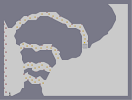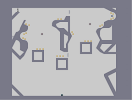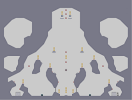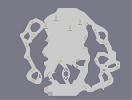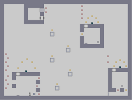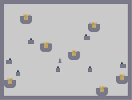Hurricane Abstuctual Caved Mighty Pirate Lasers [] [] [] [] [] [] [] [] [] [] [] [] Flying ninja

Pages: (0)

### Woagh

This map is T3H U83R 1337!

### Is He

himself?

Other than that, this is really f'ing great. awsome job, i don't watch ' so i don't know who it is though. 5/5.

very nice
cool 4,5/5

### YOUR SWORD MUST BE VERY SWIFT

One of the best i've ever seen.

I LOVE THAT SHOW

### wooh

thats really nice
couldn't do better :-)

like the silhouette effect too.
4/5!

### Really nice...

I love that silhouette effect. Not to mention the anime itself.

### AUUUUGHHH!!!

IT'S TOO GOOD!!!! OMFG!!!!1

### Dude..

Realy fucking nice.
~Mosh

^_^

...Great

I love you

### holy sh**

MUGEN!!!! LIKE MY NAME?!?!?! HOLY CRAP IVE WANTED TO DO THIS FOR SOOO LONG!! 5/5!!!